# Properties of Right Triangles: Theorems & Proofs

An error occurred trying to load this video.

Try refreshing the page, or contact customer support.

Coming up next: The Pythagorean Theorem: Practice and Application

### You're on a roll. Keep up the good work!

Replay
Your next lesson will play in 10 seconds
• 0:03 What Are Right Triangles?
• 1:51 The Pythagorean Theorem
• 3:30 Right Triangle…
• 4:56 Lesson Summary

Want to watch this again later?

Timeline
Autoplay
Autoplay
Speed

#### Recommended Lessons and Courses for You

Lesson Transcript
Instructor: Artem Cheprasov
In this lesson, you will learn about the properties of and theorems associated with right triangles, which have a wide range of applications in math and science. Specifically, we will discuss and prove the Pythagorean theorem and the right triangle altitude theorem. Let's get started.

## What Are Right Triangles?

Triangles come in many different sizes and orientations, but there's one type of triangle that stands out in particular: the right triangle. Right triangles are used in countless applications, from designing fighter jets to proving complex mathematical theorems and beyond. So what are these seemingly omnipotent geometrical structures?

A right triangle is a triangle with one of its angles measuring 90 degrees. Also, by definition, an angle measuring 90 degrees is a right angle and should be denoted by a little square, as we will see soon enough. Let's look at some examples of right triangles. Note that each of the displayed triangles has a little square to designate the right angle. This is very important since some triangles are not considered to be a right triangle because angle C is not designated as a right angle as in this image: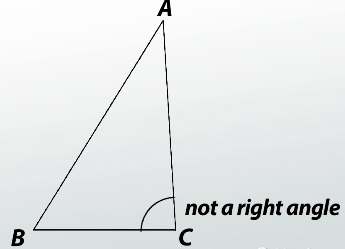An important property of right triangles is that the measures of the non-right angles (denoted alpha and beta in this figure) must add up to 90 degrees. This stems from the fact that the sum of all angles in a triangle is 180 degrees, so alpha plus beta plus 90 equals 180 degrees. Implying that, alpha plus beta equals 90 degrees.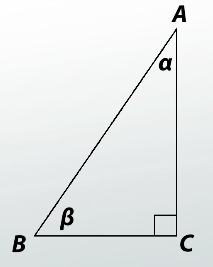By the way, angles that add up to 90 degrees are also called complementary angles, in case you read that somewhere else. The sides opposite angles alpha and beta are called legs, while the side opposite the right angle is called the hypotenuse.

With all of that out of the way, you're now ready to proceed to two important theorems that have made right triangles super famous.

## The Pythagorean Theorem

The Pythagorean theorem is named after the Greek mathematician Pythagoras, although it was also discovered independently by other ancient civilizations. It describes the relation between the two legs and hypotenuse of a right triangle. Take a look at the figure below. Note that the two legs are denoted as a and b, while the hypotenuse is denoted as c. The Pythagorean theorem states that the same of the squares of the lengths of the legs is equal to the square of the length of the hypotenuse, or more simply, a squared plus b squared equals c squared.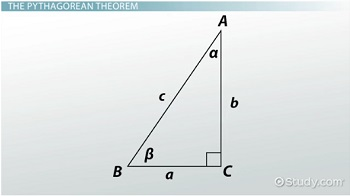Let's look at a proof of this theorem proposed by James Garfield. We start with a right triangle, duplicate it, and place it next to the original copy as shown.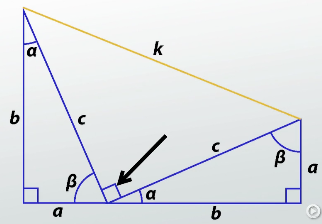Adding an extra line from the tip of angle alpha to the tip of angle beta, as shown by the orange line, we turn the whole figure into a trapezoid. Note that the angle opposite line segment k must measure 90 degrees. The formula for the area of a trapezoid is one-half height times sum of the bases. Looking at our figure, both the sum of the bases and the height are equal to a plus b, so the area of our trapezoid is equal to one-half times a plus b times a plus b.

Now, summing up the areas of the two duplicate triangles with the third triangle formed by line segment k and recalling that the area of a triangle is one-half times base times height, we have this: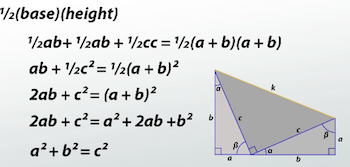To unlock this lesson you must be a Study.com Member.

### Register to view this lesson

Are you a student or a teacher?

### Unlock Your Education

#### See for yourself why 30 million people use Study.com

##### Become a Study.com member and start learning now.
Back
What teachers are saying about Study.com

### Earning College Credit

Did you know… We have over 160 college courses that prepare you to earn credit by exam that is accepted by over 1,500 colleges and universities. You can test out of the first two years of college and save thousands off your degree. Anyone can earn credit-by-exam regardless of age or education level.